###### How to Solve Word Problems Programmatically

Chapter

7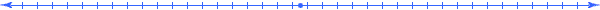Chapter 7.   Relationships

 7.1 System of Abstract Things 7.2 Relating Quantities 7.3 Defining Dimensions 7.4 Unified Quantities in Abstract Systems 7.5 Applying Abstract Systems 7.6 State of a System 7.7 Physical Systems 7.8 Comparative Systems 7.9 Business Transaction Systems 7.10 Finance Systems 7.11 Dynamic Systems 7.12 Activities and Explorations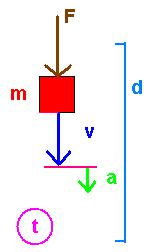7.1

#### System of Abstract Things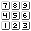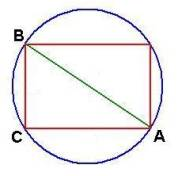System of Abstract Things:   Circle Rectangle Line Points A, B, and C

A system of abstract things contains things that have common parts or related dimensions.  Above we have an abstract system of things including of a line between two opposite corner points (A and B) of a rectangle that lie on a circle.  Even though we may describe a few initial things in a system, there usually exist many other interrelated things and subthings.  In this abstract system, we can further identify two adjacent right triangles and various arc segments.  We will also say "abstract system" to refer to a system of abstract things.

7.2

#### Relating Quantities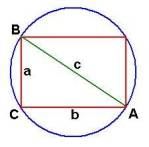####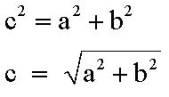The quantities a, b, and c of the dimensions SideA, SideB, and SideC of the thing TriangleABC relate to each other through the Pythagorean theorem for right triangles.  If these three quantities were to appear in a word problem, we can then use this relationship to relate on of these sides to the other two.  For instance, we may relate the third side entered into the meaning table with the other two sides.

 Qty Value Meaning Relationship q1 a [m~SideA(TriangleABC)] q2 b [m~SideB(TriangleABC)] q3 c [m~SideC(TriangleABC)] sqrt(q1^2+q2^2)

7.3

#### Defining DimensionsQty Value Meaning Relationship q1 a [m~SideA(TriangleABC)] q2 b [m~SideB(TriangleABC)] q3 c [m~SideC(TriangleABC)] sqrt(q1^2+q2^2) q4 d [m~Diameter(Circle)] q3 q5 p [m~Perimeter(Rectangle)] 2*q1+2*q2 q6 q [m~Perimeter(TriangleABC)] q1+q2+q3 q7 C [m~Circumference(Circle)] π *q4 q8 r [m~Radius(Circle)] q4/2 q9 A [m2~Area(Rectangle)] q1*q2 q10 X [m2~Area(TriangleABC)] q1*q2/2 q11 Y [m2~Area(Circle)] p*q8^2

Other relationships come from the definition of new dimensions.  In such cases, a given word (like diameter, perimeter, and circumference) determines appropriate relationships.   Notice how the relationships entered into the above meaning table come from definition formulas such as:

p=2*a+2*b

q= a+b+c

C= π * d

r= d/2

A= a*b

X= a*b/2

Y=p*r^2

Each of the entries in the meaning table illustrates an additional dimension of a system consisting of a rectangle inscribed by a circle introduced by relating it to previously defined dimension.  In essence, the expressions placed in the Relationship column of the meaning table come form the definition of the that dimension.  When formulating word problems using Unified Math®, we will take this general approach of introducing formulas as definitions of the meaning associated with the quantities.

7.4

#### Unified Quantities in Abstract Systems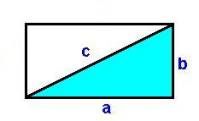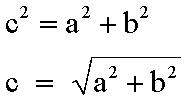a b c sqrt(a2+b2)  a+b+c 2*a+2*b a*b a*b/2 [m~Width(Rectangle)] [m~Height(Rectangle)] [m~Diagonal(Rectangle)] [m~Hypotenuse(RightTriangle)] [m~Perimeter(RightTriangle)] [m~Perimeter(Rectangle)] [m2~Area(Rectangle)] [m2~Area(RightTriangle)] Unified Quantity Expressions in a System of Abstract Things

A system of abstract things (like rectangles, triangles, and circles) can have a lot of unified quantities based on the interdependence of the various dimensions.  In this case the triangles share sides with the rectangle and this leads to a set of various related dimensions.

7.5

#### Applying Abstract Systems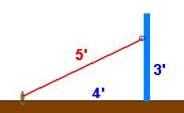Dimensions that we define and relate in a system of abstract things help us to analyze a corresponding system of real things.  For example, the relationship of the hypotenuse and the other sides of an abstract triangle can help us determine the length of a rope between a ring 3 yards up the side of a pole and a stake in the ground 4 yards away from the pole.  A meaning table can document one of many thought processes to find the length of the rope.  Suppose we did not know that the length was 5 feet.  Since the pole forms a right angle with the ground, that invokes the abstract right-triangle model with all its inherent dimensions and relationships.  So we enter our goal (c) into the meaning table and find related information.

 Qty Value Meaning Relationship q1 c [m~Length(Rope)] sqrt(q2^2+q3^2) q2 4 [m~LengthTo(Stake)] q3 3 [m~Height(PoleRing)]

On substituting the known values of the quantities we can formulate and solve the word problem:

c = sqrt(4^2+3^2) = sqrt(25) = 5.

Notice that we used the following correspondence between the dimensions of the real world situation and the dimensions of the abstract system:

 [m~LengthTo(Stake)] [m~Height(PoleRing)] [m~Length(Rope)] [m~Width(RightTriangle)] [m~Height(RightTriangle)] [m~Hypotenuse(RightTriangle)]

For generality purposes, we encourage you to use the abstract mathematical terminology (see Suggested Terminology) instead of problem specific words when building the meaning table so that you can find related dimensions and relationships more readily; in fact, programmatically.  For example, either you or a computer, could immediately introduce two new dimensions on entering something like "hypotenuse" to complete the meaning just as easily as we introduce unit conversions or undefined scaled dimensions.

 Qty Value Meaning Relationship q1 c [m~Hypotenuse(RightTriangle)] sqrt(q2^2+q3^2) q2 [m~Width(RightTriangle)] q3 [m~Height(RightTriangle)]

7.6

#### State of a System4 3 5 sqrt(42+32)  4+3+5 2*4+2*3 4*3 4*3/2 [m~Width(Rectangle)] [m~Height(Rectangle)] [m~Diagonal(Rectangle)] [m~Hypotenuse(RightTriangle)] [m~Perimeter(RightTriangle)] [m~Perimeter(Rectangle)] [m2~Area(Rectangle)] [m2~Area(RightTriangle)] a b c sqrt(a2+b2)  a+b+c 2*a+2*b a*b a*b/2

A state of an abstract system specifies a particular instance of the system.  When we formulate a model for a given word problem, we construct a particular instance of the system and use the resulting formulas to find other quantities in that particular instance.  In each given situation, we may only need a few of the many dimensions that exist in a given system.  In essence, the mathematical models developed in a meaning table describe the relationships between dimensions of real things for a given instance.

7.7

#### Physical System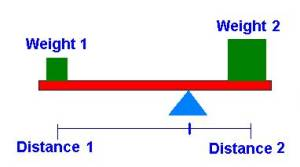Principle of Balance:   w1 * d1 = w2 * d2

A fundamental relationship discovered by the Greek mathematician Archimedes of Syracuse (around 250 BC) describes the relationship between things on a balance placed at specific distances away from the center of the balance.   The following unified quantities and their relationship characterize this system:

 Qty Value Meaning Relationship q1 w1 [kg~Weight(Thing1)] q2 w2 [kg~Weight(Thing2)] q3 d1 [m~LengthFromCenter(Thing1)] q4 d2 [m~LengthFromCenter (Thing2)] q1*q2/q3

7.8

#### Comparative SystemsHow do we determine if one thing is "greater" than another?  Is the rectangle on the left "greater" than the one on the right?  It depends on our choice of dimensions that we compare.  If we use the dimension height to determine the meaning of "greater" then the rectangle on the left is larger, even though it appears thinner with far less area.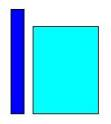Comparing Two Things

Many words in the English language refer to comparisons, many of which have opposites: thinner-smaller, colder-hotter, heavier-lighter, top-bottom, etc.  What does it mean to be on "top"?  The answer "a greater vertical length from the earth" implies mathematical measurements and comparisons.  These words translate into the abstract concepts of "greater than" (>) and "less than" (<).  Their use in word problems usually invokes a measure of how much greater or how much less than, words that indicate adding and subtracting.  We consider all such comparative words as mathematical vocabulary words.  Such words usually lead to relationships involving either an addition or a subtraction.  Consider the following phrases:

 sue is 2 inches taller than john the box weighs 10 pounds lighter than the lamp today's temperatures is 3 degrees higher than normal

The following unified quantities and their relationship characterize these phrases:

 Qty Value Meaning Relationship q1 j [in~Height(John)] q2 s [in~Height(Sue)] q1+2 q3 L [lb~Weight(Lamp)] q4 B [lb~Weight(Box)] q3-10 q5 N [°F~Temperature(Normal)] q6 N+3 [°F~Temperature(Today)]

Notice in the last line that we entered the relationship as a mathematical expression in the value column instead of the Relationship column.  Indeed we can enter relationships on either side of the meaning table, but usually only simple ones (like these examples) in the value column.   We could have done the same for q4  with B replaced by L-10; and the same for q2  with s replaced with j+2.  This approach reduces the number of variables introduced in the meaning table.

Comparative systems may be the first type of systems that we encounter with mathematics.  Babies can feel comparisons.  They close their eyes in brighter light or jerk back when exposed to hotter temperatures.

7.9

#### Business Transaction SystemsRelationships in a Business Transaction

In the above diagram, the quantities on the right (wc, rc, sp, mp) are placed on a line (oriented vertically) to indicate the corresponding relationships between them; namely, wc is less than rc, rc is less than sp, and sp is less than mp.   The quantities on the left (sd, rp, wp) are defined as the differences between their adjacent values.   This illustrates how we can define the dimensions associated with a typical business transaction by their relationships.  For example, we define a sales discount as the difference between the marked price and the sales price.  Such definitions can be used in a meaning table as follows.

 Qty Value Meaning Relationship q1 mp [dol~Marked Price(Thing)] q2 sp [dol~Sale Price(Thing)] q3 rc [dol~Retail Cost(Thing)] q4 wc [dol~Wholesale Cost(Thing)] q5 sd [dol~Sales Discount(Thing)] q1-q2 q6 rp [dol~Retail Profit(Thing)] q2-q3 q7 wp [dol~Wholesale Profit(Thing)] q3-q4 q8 a [ins~Occurrence(Thing)] q9 tc [dol~Total Cost(Thing)] q8*q2

The last two dimensions define how the total cost depends on the number of items and the sales price of those items.

7.10

#### Finance Systems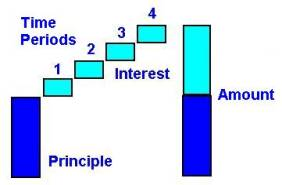Relationships in a Simple Interest Loan

This diagram illustrates the relationships between the dimensions involved in a simple interest loan.

The interest dimension associated with a simple interest finance model is usually given in terms of a fraction of the principle paid over t time periods.

 Qty Value Meaning Relationship q1 p [dol~Principle(Loan)] q2 I [dol~Interest(Loan)] q1*q3*q4 q3 t [month~Time(Thing)] q4 r [dol~Interest(Loan)] /([dol~Principle(Loan)]* [month~Time(Thing)]) q5 a [dol~Amount(Loan)] q1+q2

7.11

#### Dynamic Systems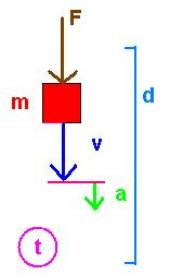d [m~Distance(FallingThing)] t [s~Time(FallingThing)] v [m/s~Velocity(FallingThing)] a [m/s2~Acceleration(FallingThing)] m [kg~Mass(FallingThing)] F [N~Force(FallingThing)] A Dynamic System

The word "dynamic" implies that things are in motion.  Sir Isaac Newton, one of the inventors of calculus, explored the relationships between the dimensions associated with things attracted to each other by gravity.  Today we use his name to specific a unit of force; so 20 N (read twenty newtons) specifies an amount of force.  By definition N=kg*m/s2.  He found that the acceleration (a) that pulls a thing toward the earth is a constant of proportionality between the Force (F) exerted on the thing and the mass (m) of the thing.  So for gravity, F=m*a.  This and other relationships characterize a dynamic system:

 Qty Value Meaning Relationship q1 d [m~Distance(FallingThing)] q2 t [s~Time(FallingThing)] q3 v [m/s~Velocity(FallingThing)] q1/q2 q4 a [m/s2~Acceleration(FallingThing)] q3/q2 q5 m [kg~Mass(FallingThing)] q6 F [N~Force(FallingThing)] q5*q4

Take caution though, the relationships for the quantities q3 and q4 only apply if their corresponding ratios represent constants of proportionality; otherwise we use the calculus concept of a derivative to define the relationship.   Similarly, the relationship for the q6 only applies if the mass dimension and the acceleration dimension are independent (have a constant horizon function as we will discuss later); otherwise we use the calculus concept of an integral to define the relationship.

7.12

#### Activities and ExplorationsActivities:

 List relationships Develop a list of relationships from the explorations below.   Get Physical Explore relationships in nature (notice how many things are proportional) http://www.walter-fendt.de/ph11e/

Explorations:

 Explore Math Relationships http://www.math.com/tables/index.html (various math categories)   Explore Geometry Relationships http://mathforum.org/dr.math/faq/formulas/  (geometry formulas)   Explore Physics Relationships http://physics.nist.gov/cuu/Units/units.html  (SI formulas)

Exercises:

 Do Related Problems In Your Textbook

Copyright © 2004  Dr. Ranel E. Erickson